﻿

### Conformally recurrent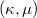$( \kappa,\mu)$-contact manifolds

#### Abstract

In this paper it is shown that a conformally recurrent$(\kappa,\mu)$-contact space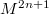$M^{2n+1}$,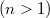$(n>1)$ is locally isometric to either (i) unit sphere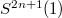$S^{2n+1}(1)$ or (ii)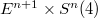$E^{n+1} \times S^{n}(4)$

DOI Code: 10.1285/i15900932v28n2p207

Keywords: Confomally recurrent; Contact metric manifolds; tangent sphere bundle

Classification: 53C15; 53D15

Full Text: PDF